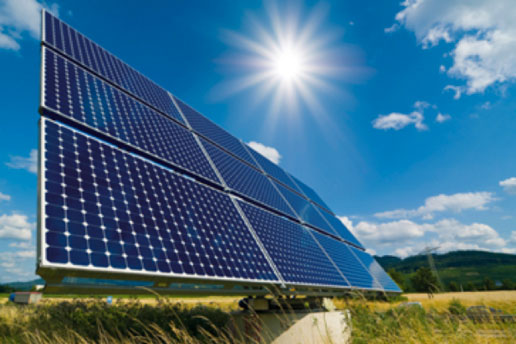Verification of thermoemf with temperature of hot junctionLet us suppose two junctions of a thermocouple are kept in a melting ice (0o C) so they become a junction and θc = 0o C, where θc is temperature of cold junction. Now, one junction is heated so it becomes a hot junction. If the temperature of hot junction is increase gradually, the thermoemf also increases continuously and becomes maximum at certain temperature of hot junction. The temperature of hot junction at which thermoemf becomes maximum is called neutral temperature and is denoted by θn. If the temperature of hot junction after neutral temperature is increased, the thermoemf decreases continuously and becomes zero at certain temperature of hot junction. The temperature at which thermoemf becomes zero is called temperature of inversion and is denoted by θi. Again, if the temperature of hot junction is increased beyond from temperature of inversion, the thermoemf starts to increase but in opposite direction. The variation of thermoemf with temperature of hot junction can be sown in following graph.From the graph it is seen that,

θn -  θc  =  θi - θn

n  =  θc + θi

θn  =  ( θc + θi )/2

i.e., neutral temperature is the arithmetic mean of temperature of cold junction and temperature of inversion.

Theory:

We know that there are a large number of free electrons in metals. The number of free electrons per unit volume is different to different metals. When two wires of different metals are joined to form a junction, the diffusion of electrons take place at the junction and the potential called a contact potential is developed at the junction. When two junctions of a thermocouple are at same temperature, the same contact potential developed and hence the contact potential does not differ and becomes zero i.e. no current flows. But when one junction is made hot and the other cold, there is more contact potential at hot junction than that at cold junction, and hence the contact potential called thermoemf is developed across the junction so called flows.